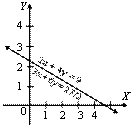# Mathematics

### Chapter : Linear Equation In Two Variable

#### Solutions of Pair of Lines in Two Variable

There are three types of solutions of Pair of Lines in Two Variable, these are

• Unique solution
• Infinitely many solutions
• No solution.

(A) Situation of Consistent: If a system of simultaneous linear equations has at least one solution then the system is said to be consistent.

(i) Consistent equations with unique solution : The graphs of two equations intersect at a unique point. For example. Consider the pair of equation of lines x + 2y = 4 and 7x + 4y = 18.The graphs of pair of lines of these equations intersect each other at the point (2, 1) i.e., x = 2, y = 1. Hence, the equations are consistent with unique solution.

(ii) Consistent equations with infinitely many solutions : The graphs (lines) of the two equations will be coincident. For example. Consider the pair of equations 2x + 4y = 9 ⇒ 3x + 6y = 27/2The graphs of the above equations coincide. Coordinates of every point on the lines are the solutions of the equations. Hence, the given equations are consistent with infinitely many solutions.

(B) Inconsistent Equation : If a system of simultaneous linear equations has no solution, then the system is said to be inconsistent.
No Solution : The graph (lines) of the two equations are parallel. For example. Consider the pair of equation of lines are 4x + 2y = 10 and 6x + 3y = 6The graphs (lines) of the given equations are parallel. They will never meet at a point. So, there is no solution. Hence, the equations are inconsistent.

 S. No Graph of Two Equations Types of Equations 1. Intersecting Lines Consistent, With Unique Solution 2. Coincident Consistent with number of infinite solutions 3. Parallel Lines Inconsistent or No Solution is found